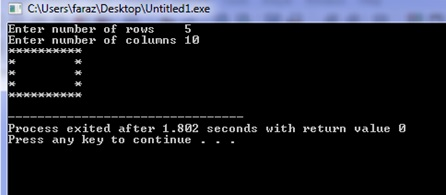# C program for 'Hollow Rectangle' Pattern

In this c program, we are going to read total number of rows, columns and print a 'Hollow Rectangle'.
Submitted by Shamikh Faraz, on February 14, 2018

In this program we will learn, how we can print hollow rectangle using ‘asterisks’ and ‘loops’.

C program

﻿

```#include<stdio.h>
int main(){
int columns, rows, a, b;

printf("Enter number of rows\t");
scanf("%d", &rows);
printf("Enter number of columns\t");
scanf("%d", &columns);

/*this loop increase number of rows */
for(a = 0; a < rows; a++)
{
/*this loop increase number of columns */
for(b = 0; b < columns; b++)
{
/* this puts the star on boudaries */
if(a==0 || a==rows-1 || b==0 || b==columns-1)
printf("*"); //prints the star
else
printf(" ");
/* leaves the middle columns of rectangle blank*/
}
printf("\n");
}
return 0;
}
```

Output